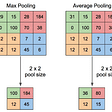# ReLU (Rectified Linear Unit) linear or non-linear, that is the question…

The activation function is an integral part of a neural network. It is used to activate the neurons or nodes throughout the layers. The activation function used in the hidden layers mainly controls the learning of a module. Depending upon the values of the output of the activation function, the weights and biases are further improved in backpropagation. The output activation function decides which kind of output the model or network will produce whether it is binary classification, multiclass classification or multilabel classification it totally depends upon the activation function we choose in the output layer.

## Problems with Linear activation function

A linear activation function is helpful in case of regression problems where the model has to predict a real value (not just 0/1) like to predict the runs scored in the match or stock price. But in a convolutional neural network or multilayer perceptron network, it won’t work. In this network linear activation function will be more or less useless as the output of a node will be the linear function of the input. This function will not help the network to learn from the data. Hence the non-linear functions like Sigmoid, tanh functions are better options.

## But is sigmoid good enough?

Very often deep networks are used to train a model instead of a shallow network. In any network activation function is being used and during backpropagation, the derivatives of nodes are calculated. Now for a deeper network, a problem named “vanishing gradient” may arrive. The activation function like sigmoid squishes the input from a large range to (0,1) range.

`                          f(x)=1/(1+e^(-x))`

Hence the large change in the input also causes a very tiny change in the output of the function. Hence, the gradient descent will be close to 0. For shallow networks, this may not be a problem as we don’t have so many layers. But for a deep network, as we iterate over the layers during backpropagation and multiply the output of the activation function with the weights, the gradient converges to 0.

## Advantage of ReLU over Sigmoid

For ReLU (Rectified Linear Unit) the curve is bent, not curved hence the derivative is not defined where the function is bent. That is a problem because Gradient Descent needs derivatives for all points and from those values, we have to estimate the weights and biases for the further improvement of the model/network.

## Modification in ReLU

Though ReLU can solve the problem of vanishing gradients, it comes up with another one called Dead ReLU. This happens because of the discontinuity of the ReLU function. The derivative of ReLU is 1 when value>0 and derivative is 0 when value<0 but the derivative at value=0 is unknown. Hence, a modified function is nowadays used which is known as LReLU. The change in the equation is we will use a value close to 0 instead of 0.

`                           f(x)=max(0.01*x,x)`

## Conclusion

Though in many places we see that ReLU is being used as a linear function, it is actually not. A linear function allows you to divide the feature plane into halves but the non-linearity of ReLU can create arbitrary shapes in the feature plane.

## References

1.https://datascience.stackexchange.com/questions/26475/why-is-ReLU-used-as-an-activation-function

--

--

--

## A Summary of Recent SDA Contributions towards improving Entity Disambiguation, Linking and…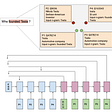## Dissecting Optimization Algorithms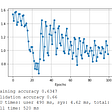## Curated list of Outlier Detection Resources## What exactly does CNN see?## Artificial Neural Network Explained with an Regression Example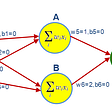## My MangaGAN: Building My First Generative Adversarial Network## The Big Three (Approaches to ML)## Sawon

Always eager to learn more about new technologies and finding a way to make a better future with AI.

## Invasive Species Monitoring: Using a Convolutional Neural Network to identify hydrangeas## Multiple Inputs & Multiple Outputs in a Neural Network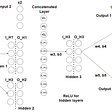## Pooling layers in Neural nets and their variants